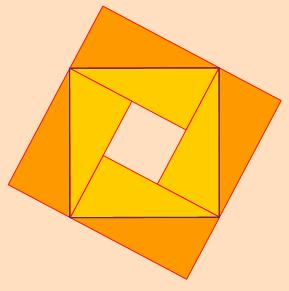#### You may also like### Doodles

Draw a 'doodle' - a closed intersecting curve drawn without taking pencil from paper. What can you prove about the intersections?### Russian Cubes

I want some cubes painted with three blue faces and three red faces. How many different cubes can be painted like that?### Picture Story

Can you see how this picture illustrates the formula for the sum of the first six cube numbers?

# Zig Zag

### Why do this problem :

There is an important configuration of four congruent right-angled triangles which make a square in two ways. Either the sides of the square are formed from each triangle's hypotenuse, or from putting together the two lengths adjacent to the right angle in the triangle. This problem uses two of the four matching points from each configuration. The other two points are not shown and so exploring this problem will draw students into discovering this arrangement and also provides experience in how a situation may sometimes present as only part of a more meaningful or useful 'whole'.### Possible approach :

Ask students to guess whether the four points indicated do or do not lie on a straight line, especially once the triangles are free to move as a general right-angled triangle.

There is scope for an activity to draw accurately the configuration shown in the problem, but with right-angled triangles which students form for themselves.

Alternatively use a Dynamic Geometry package, but in either case move the group into a discussion about whether these four points form one straight line or not.

The aim, as the exploration continues, is to cause some high-energy discussion shot-through with geometric reasoning, where students challenge each other to say why they are sure that this is one line. And as they do that the configuration illustrated above is discovered along the way.

### Key questions :

• What does this diagram show ?

• Are the four points aligned or just close to it, and would that answer change if the arrangement used right-angled triangles of another proportion ?

• How can you be sure of that ?

• What perspective would you try to share with others to help them see and enjoy the relationships within this arrangement ?

### Possible extension :

Squirty is a good companion problem if this activity is pursued using standard geometric construction with straight edge and compasses.

### Possible support :

Less able students will gain much by making and then using congruent triangles to make patterns based on the configuration shown above.